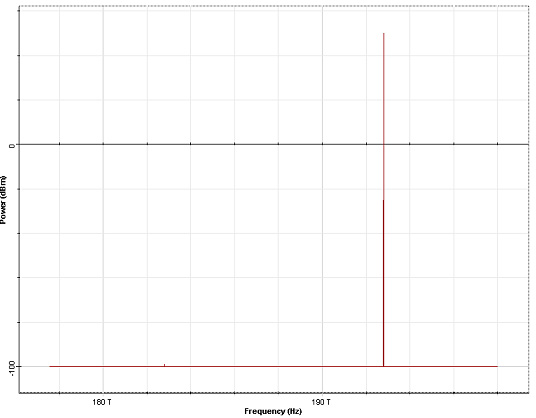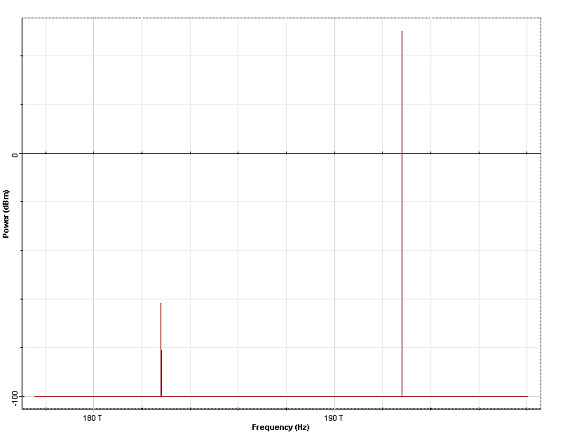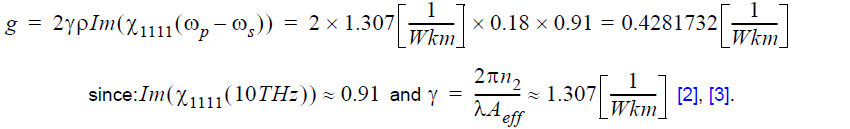This lesson demonstrates the light amplification caused by the stimulated Raman scattering effect. The layout and its global parameters are shown in Figure 1.

Figure 1: Layout and global parameters

Figure 2 shows the setup for the optical fiber component.

Figure 2: Setup parameters

The spectrum of the input signal consists of a strong pump monochromatic wave (100 W) at 1550 nm and a weak (-99 dBm) Stokes wave at 1640 nm (10 THz Stokes shift) (see Figure 3).Figure 3: Input signal spectrum

Figure 4 gives the output spectrum. The weaker (low frequency) spectral component is amplified and the gain is G=99-61.7=37.3 dB.Figure 4: Output signal spectrum

Raman gain coefficient is given  by:The Raman gain (in dB) is then G =  10log(exp(gPL)) = 37.2dB, where P is
the pump power and L is the fiber length.

References
G. P. Agrawal, “Nonlinear fiber optics”, Academic press, 3rd edition, 2001.

R. W. Hellwarth, Prog. Quant. Electr. 5, 1 (1977).

P. Tchofo Dinda, G. Millot, and S. Wabnitz, JOSA B, 15, 1433, (1998).